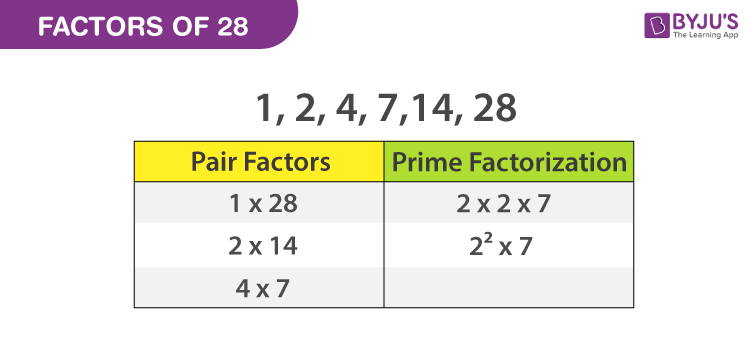# Factors of 28

The factors of  28 are the numbers that can evenly divide 28. These factors are 1,2,4,7,14 and 28. When we divide 28 by any of its factors, then the remainder will be equal to 0 and the quotient will be a natural number.

Suppose, 28 divided by 7 is equal to 4, thus 7 is one of the factors of 28.

28/7 = 4

Similarly, the pair factors are the set of numbers that on multiplication produces the original number.  For example, 1 and 28 both are the factors of real number 28, since, 1 multiplied by 28 is equal to 28 itself.

1 x 28 = 28

Let us learn how to find the factors and pair factors of twenty-eight using factorisation and division method at BYJU’S.## What are the Factors of 28?

Factors of 28 are the natural numbers that can divide the original number evenly, without leaving any remainder.

 Factors of 28 1, 2, 4, 7, 14, and 28

## Pair Factors of 28

To find the pair factors, multiply the two numbers in a pair to get the original number as 28, such numbers are as follows

1 × 28 = 28, then (1, 28) is a pair factor of 28.

2 × 14 = 28, (2, 14) is a pair factor of 28

4 × 7 = 28, (4, 7) is a pair factor of 28

Therefore, the positive pair factors are (1, 28), (2, 14), and (7, 4).

To find the negative pair factors of 28, then proceed with the following steps

-1 × -28 = 28, then (-1, -28) is a pair factor of 28.

-2 × -14 = 28, (-2, -14) is a pair factor of 28

-4 × -7 = 28, (-4, -7) is a pair factor of 28

Therefore, the negative pair factors are (-1, -28), (-2, -14), and (-7, -4).

### How to Find the Factors of 28?

To find the factors of a number, we need to check that by which factor the original number is completely divisible.

In case of number 28, we will see, the factors that can divide 28, evenly.

• 28 ÷ 1 = 28
• 28 ÷ 2 = 14
• 28 ÷ 4 = 7
• 28 ÷ 7 = 4
• 28 ÷ 14 = 2
• 28 ÷ 28 = 1
 Factors of 28 1, 2, 4, 7, 14, and 28

Apart from these factors, if we try to divide 28 by any other natural number, then the resulting quotient will be a fraction.

Example: 28 ÷ 3 = 9.33.. (fraction)

## Prime Factors of 28 By Division Method

The number 28 is a composite and it should have prime factors. Now let us know how to calculate the prime factors.

• The first step is to divide the number 28 with the smallest prime factor, say 2.

28 ÷ 2 = 14

• Again divide 14 by 2 and the process goes on.

14 ÷ 2 = 7

• Now, if we divide 7 by 2, we get a fractional number, that cannot be a factor. So, continue with the next prime factor, say 7

7 ÷ 7 = 1

Finally, we got the number 1 at the end of the division process. So that we cannot proceed further. So, the prime factors of 28 are written as 2 × 2 × 7 or 22 x 7, where 2 and 7 are the prime numbers.

## Video Lesson on Prime Factors## Solved Examples

Q.1: If there are 28 candies to be distributed among 4 children. How many candies does each child get?

Solution: Given,

Number of candies = 28

Number of children = 4

Each child will get = 28/4 = 7 candies

Q.2: If there are 14 chalks in one box and the number of boxes is 2, then how many total chalks are there in the boxes?

Answer: Given, each box has 14 chalks

Number of boxes = 2

Therefore, total number of chalks = 14 x 2 = 28

Q.3: What are the common factors of 28 and 56?

Answer: The factors of 28 are 1, 2, 4, 7, 14, 28 and factors of 56 are 1, 2, 4, 7, 8, 14, 28, 56. Therefore the common factors of 28 and 56 are 1, 2, 4, 7, 8, 14, 28.

Register with BYJU’S – The Learning App to know the factors of 28 and also learn the factors and prime factors of other numbers with examples.

## Frequently Asked Questions – FAQs

Q1

### What are the total factors of 28?

The factors of 28 are 1, 2, 4, 7, 14, and 28.
Q2

### Are multiples and factors of 28 same?

Multiples and factors of 28 are different and not the same. Factors of 28 are finite values but multiples of 28 are infinite.
Q3

### What are the multiples of 28?

The first 10 multiples of 28 are 56, 84, 112, 140, 168, 196, 224, 252, 280, 308.
Q4

### What are the prime factors of 28?

The prime factors of 28 are 2, 2, and 7.
Q5

### What are common factors of 28, 32 and 48?

Factors of 28 = 1, 2, 4, 7, 14, 28
Factors of 32 = 1, 2, 4, 8, 16, 32
Factors of 48 = 1, 2, 3, 4, 6, 8, 12, 16, 24, 48
Therefore, the common factors of 28, 32 and 48 are 1, 2 and 4.
 Links Related to Factors Factors of 8 Factor of 36 Factors of 48 Factors of 18 Factors of 24 Factors of 25 Factors of 42 Factors of 60 Factors of 35 Factors of 81 Factors of 75 Factors of 56
Quiz on Factors of 28